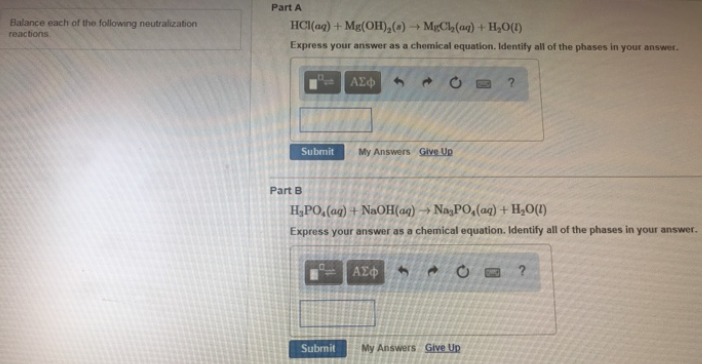# Problem: Balance each of the following neutralization reactions. Part AHCl (aq) + Mg(OH)2 (s) → MgCl2 (aq) + H2O (l) Express your answer as a chemical equation. Identify all of the phases in your answer. Part BH3PO4 (aq) + NaOH(aq) → Na3PO4(aq) + H2O(l) Express your answer as a chemical equation. Identify all of the phases in your answer.

###### FREE Expert Solution
79% (472 ratings)###### Problem Details

Balance each of the following neutralization reactions.

Part A

HCl (aq) + Mg(OH)2 (s) → MgCl2 (aq) + H2O (l)

Part B

H3PO4 (aq) + NaOH(aq) → Na3PO4(aq) + H2O(l)